# Root Mean Square Error (RMSE) in Python and Machine Learning

Root Mean Square Error (RMSE) is a standard way to measure the error of a model in predicting quantitative data.

RMSE in Machine Learning is used to calculate the transformation between values predicted by a model and actual values. Using RMSE, we can easily find the difference between a model parameter’s estimated and actual values.

## Formula of RMSE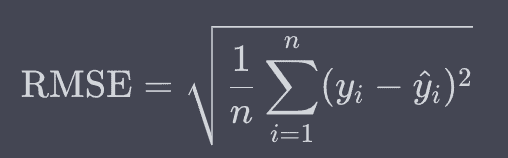Where,

1. yi: It is the actual value.
2. ^yi: It is the predicted value.
3. n: It is the number of observations.

## Real-time examples of RMSE

1. Weather Forecasting
2. Stock Market Prediction
3. Real Estate Price Prediction

## How to Calculate RMSE for a DataSet [Machine Learning Project]

To understand RMSE (Root Mean Square Error) comprehensively, we will go through the following steps:

1. Dataset Selection: We will begin by selecting a dataset, preferably a regression dataset since RMSE is typically used for regression problems.
2. Data Exploration: We will understand the dataset’s structure, missing values, and general statistics.
3. Data Preprocessing: We will clean the dataset, handle missing values, and perform feature scaling if necessary.
4. Model Training: We will split the dataset into training and testing sets and then train a regression model.
5. Evaluation: We will use RMSE to evaluate the model’s performance on the test set.
6. Visualization: We will visualize the actual versus predicted values to understand the errors’ distributions.

### Install required libraries

If you have not installed libraries, then you can install pandas, numpy, matplotlib, seaborn, and scikit-learn libraries using pip:

``pip install pandas numpy matplotlib seaborn scikit-learn``

### Step 1: Data Selection and Exploration

We will use the realtor-data.csv file for this project. You can download the dataset from here.

To import external datasets and read them as a DataFrame in Python, use the Pandas.read_csv() method.

``````import pandas as pd

# Display the first few rows of the dataset
``````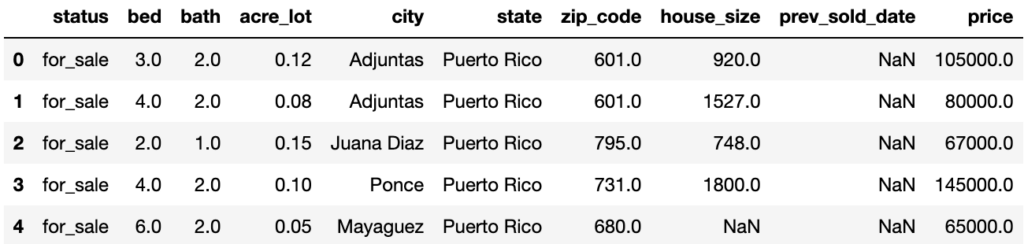Our target variable for regression will be “price.”

Next, we should check for any missing values in the dataset, understand the data types of each column, and get some general statistics about the dataset

``````# Check for missing values
missing_values = df.isnull().sum()

# Check the data types of each column
data_types = df.dtypes

# General statistics of the dataset
statistics = df.describe()

print(missing_values, data_types)
``````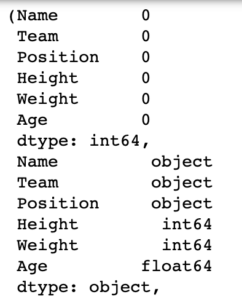### Step 2: Data Preprocessing

Given the structure of the dataset, we will perform the below preprocessing steps:

1. Handle missing values.
2. Convert categorical variables to numerical ones (if needed for our model).
3. Handle outliers and split the data into training and testing sets.

#### 2.1: Handle the missing values

1. For numerical columns: Fill missing values with the median of that column (since the median is less sensitive to outliers).
2. For categorical columns: Fill missing values with that column’s mode (most frequent value).
3. Drop the prev_sold_date column due to the high number of missing values.
``````# Drop the 'prev_sold_date' column
df.drop(columns=['prev_sold_date'], inplace=True)

# Fill missing values for numerical columns with their median
numerical_cols = ['bed', 'bath', 'acre_lot', 'zip_code', 'house_size', 'price']

for col in numerical_cols:
median_value = df[col].median()
df[col].fillna(median_value, inplace=True)

# Fill missing values for categorical columns with their mode
categorical_cols = ['city']
for col in categorical_cols:
mode_value = df[col].mode()
df[col].fillna(mode_value, inplace=True)

# Check again for missing values
df.isnull().sum()
``````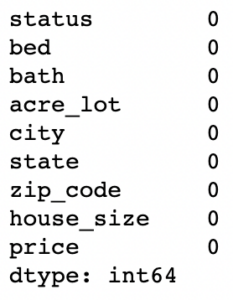#### 2.2: Convert categorical variables to numerical ones

We can use one-hot encoding for the status and state columns since they likely have limited unique values.

However, for the city column, considering that there might be many unique cities, using one-hot encoding might result in a large number of columns. Instead, we can convert the city column into numerical values based on the mean price in each city.

``````# One-hot encode the 'status' and 'state' columns
df = pd.get_dummies(df, columns=['status', 'state'], drop_first=True)

# Convert 'city' to numerical based on the mean 'price' in each city
city_price_mean = df.groupby('city')['price'].mean().to_dict()
df['city'] = df['city'].map(city_price_mean)

# Check the transformed dataset
``````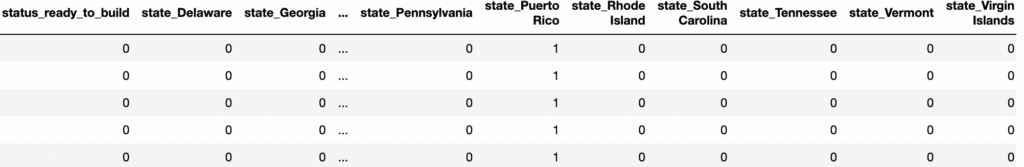#### 2.3: Handle outliers and Split the data into training and testing sets.

Outliers can have a strong effect on regression models, so it’s essential to manage them.

We will use a simple method to detect outliers: For each numerical column, values exceeding 1.5 times the interquartile range (IQR) from the 1st and 3rd quartiles will be considered outliers.

We will handle outliers by capping values at certain thresholds.

We will cap values at the 99th percentile for each column to retain most data and avoid extreme values.

Let’s cap these values and split our data into training and testing sets.

``````from sklearn.model_selection import train_test_split

cols_to_visualize = ['bed', 'bath', 'acre_lot', 'house_size', 'price']

# Cap values at the 99th percentile for each column to handle outliers
for col in cols_to_visualize:
threshold = df[col].quantile(0.99)
df[col] = df[col].clip(upper=threshold)

# Split the data into training and testing sets

# Features (X) and target (y)
X = df.drop(columns='price')
y = df['price']

# Split the data (80% train, 20% test)
X_train, X_test, y_train, y_test = train_test_split(X, y, test_size=0.2, random_state=42)

X_train.shape, X_test.shape
``````

Output

``((723972, 24), (180994, 24))``

### Step 3: Model Training

We will train a regression model on our training data. For this tutorial, we will use a simple linear regression model from Scikit-learn.

``````from sklearn.linear_model import LinearRegression

# Initialize the linear regression model
lr_model = LinearRegression()

# Train the model on the training data
lr_model.fit(X_train, y_train)

# Predict on the training and testing sets
y_train_pred = lr_model.predict(X_train)
y_test_pred = lr_model.predict(X_test)

y_train_pred[:5], y_test_pred[:5] ``````

Output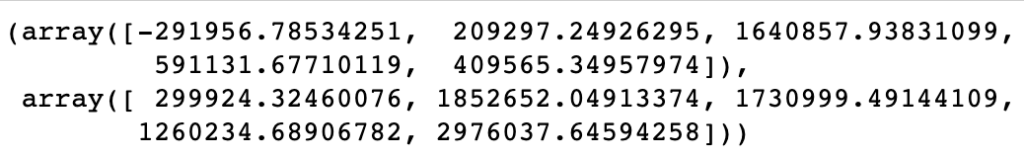### Step 4: Evaluation using RMSE

Now that we have our model’s predictions on both the training and testing sets, we can evaluate its performance using the Root Mean Square Error (RMSE).

Lower RMSE values suggest better model performance. However, comparing RMSE values between the training and testing sets is essential to check for overfitting.

If the RMSE for the training set is significantly lower than the RMSE for the test set, it might indicate that our model is overfitting the training data.

``````from sklearn.metrics import mean_squared_error
import numpy as np

# Calculate RMSE for training and testing sets
rmse_train = np.sqrt(mean_squared_error(y_train, y_train_pred))
rmse_test = np.sqrt(mean_squared_error(y_test, y_test_pred))

rmse_train, rmse_test
``````

Output

``(745954.5184758339, 744750.0479636705)``

The RMSE values are:

1. Training RMSE: \$745,954.52
2. Testing RMSE: \$744,750.05

The RMSE values for both the training and testing sets are pretty close, which is a good sign, indicating that the model is not overfitting the training data.

However, the magnitude of the RMSE is relatively high, suggesting that our model’s predictions can be off by an average of around \$745,000. This might be acceptable, depending on the specific use case, the average property price, and other factors.

### Step 5: Visualization of RMSE

To get a clearer picture of our model’s performance, it’s helpful to visualize the actual vs. predicted prices.

We will create a scatter plot for this purpose. If our model were perfect, all points would lie on a straight 45-degree line (y=x line).

Let’s visualize the actual vs. predicted prices for the test set.

``````plt.figure(figsize=(10, 6))
plt.scatter(y_test, y_test_pred, alpha=0.5)
plt.plot([0, max(y_test)], [0, max(y_test_pred)], color='red') # 45-degree line
plt.xlabel('Actual Prices')
plt.ylabel('Predicted Prices')
plt.title('Actual vs. Predicted Prices')
plt.grid(True)
plt.show()
``````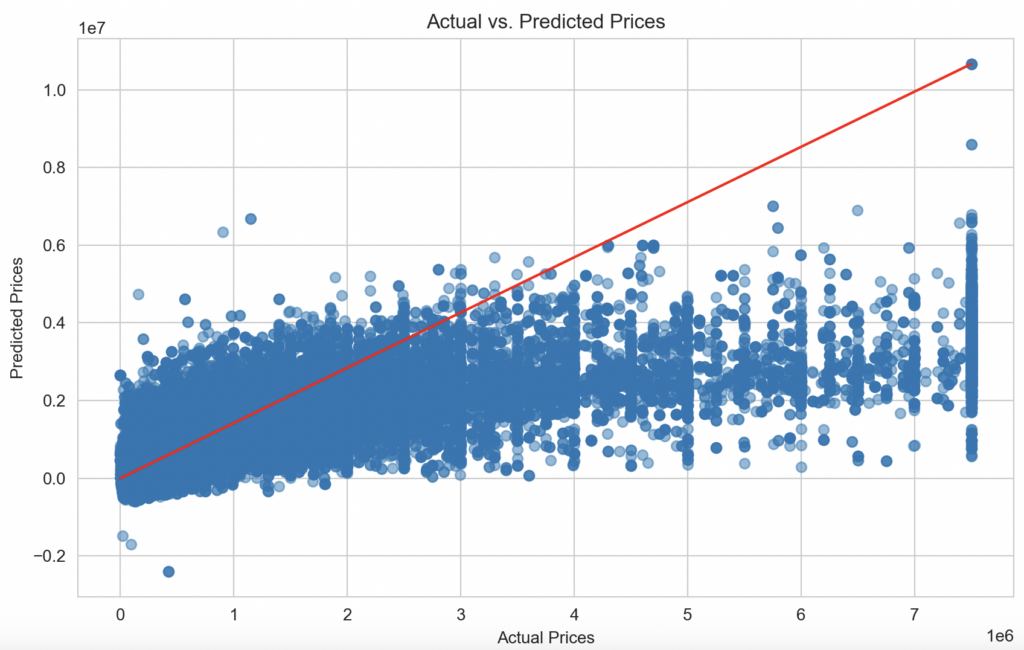The scatter plot displays the test set’s actual versus predicted property prices.

The red line represents the ideal scenario where the predicted price matches the actual price.

While many points are close to this line, indicating good predictions, we can also observe some scatter, especially for higher-priced properties. This suggests that our model has room for improvement, especially for predicting properties in the higher price range.

## Conclusion

To summarize, we:

1. Explored and preprocessed the dataset.
2. Trained a linear regression model.
3. Evaluated the model using RMSE.
4. Visualized the actual vs. predicted prices.

This real-time project serves as a basic introduction to regression modeling and RMSE.

This site uses Akismet to reduce spam. Learn how your comment data is processed.